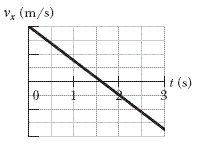# Dated box of dates, incline ramp, find normal force!

royblaze

## Homework Statement

A dated box of dates, of mass 5.00 kg, is sent sliding up a frictionless ramp at an angle of θ to the horizontal. The figure below gives, as a function of time t, the component vx of the box's velocity along an x axis that extends directly up the ramp. (The vertical axis is marked in increments of 1.5 m/s.) What is the magnitude of the normal force on the box from the ramp?Note: Does the notation of v having the subscript x have anything to do with my error? I'm assuming that the x is redundant, since the velocity's directed up the ramp entirely...right?

## Homework Equations

I'm assuming Newton's second law is in effect here?

## The Attempt at a Solution

My roommate and I both tried this question. We got an answer around 48 each and every time, and the question answer is not given (it's a input-answer-and-we-tell-you-if-it's-wrong-or-right website).

I drew a ramp and stuff, writing the force vectors all over the place and determined that at the box's highest point on the ramp, the net forces would equal zero (my roommate said that this is wrong, but I still got the same answer as he did).

From the graph given, I found the deceleration of the box to be ~1.875 m/s2. From here, I have no idea where to go. I thought you needed to find theta, but my roommate said you could also use Pythag's theorem. We've gotten ~48 about four times now.

We worked out a triangle, with hypotenuse Fw = mg, and the x-component of Fw = mgsinθ, the y-component of Fw = mgcosθ.

Solving with Pythag's, we have

sqroot(9.82 - 1.8752) = normal acceleration

We then multiplied that normal acceleration by the mass and obtained out ~48 answer.

Our answers so far (that we submitted and the machine told us we were wrong): 47.96873982, 48.09479572

Any help is appreciated! Sorry if I did something wrong, this is my first post...:shy:

## Answers and Replies

JaredJames
You get the deceleration from the graph. Your value is incorrect.

Note that the velocity changes direction - which means the object slid up the ramp, stopped and came back down. So you really should only use the time up to the point it stopped (1.5s) and the change in velocity for that time is (2). Which gives an acceleration of 2/1.5=1.33....m/s2. (Acceleration = change in velocity / time)

With that you can get the decelerating force using F = ma.

You now have the force down the ramp and you have the force straight down using F = mg.

Draw those two on the diagram with the normal as the final part of a triangle.

Now, the decelerating force is A, weight is H. Using those two with SOH CAH TOA, you can get the angle of the ramp theta and then you can get the normal force.

Last edited:
royblaze
You get the deceleration from the graph.

With that you can get the decelerating force using F = ma.

You now have the force down the ramp and you have the force straight down using F = mg.

Draw those two on the diagram with the normal as the final part of a triangle.

Now, the decelerating force is A, weight is H. Using those two with SOH CAH TOA, you can get the angle of the ramp theta and then you can get the normal force.

Okay, so:

Deceleration = 1.875
Mass = 5kg

Framp = 5 * 1.875 = 9.375 N, down the ramp
Fdue to gravity = 5 * 9.8 = 49 N, straight down

So, I have the hypotenuse and one side of the triangle, and I'm looking for the last side using SOHCAHTOA:

Can't I just resolve the Pythagorean Tirangle?

492 = 9.3752 + x2, where x is the normal force

x= 48.09479572 N

OR:

mgsin(theta) = Framp, and theta = 11.03020913 degrees.

mgcos(theta) = Fnormal, and the answer is 48.09479572 N.

Both of these answers are wrong according to the site.

Did I do it wrong?

JaredJames
EDIT: Error noted.

Last edited:
royblaze
The question states that the y-axis is marked by 1.5 m/s for every tick. That's why my deceleration is different.

For the numbers you used, it would actually be 3/1.5 = 2 m/s2...
Which isn't too far off from 1.875. I also used a deceleration of 2 in the above equations and got 47.96873982 N, which is the wrong answer.

I'm lost now on what to do... :(

Last edited:
JaredJames
I get 47.97N.

I'd recommend you don't answer so accurately just to try it, but otherwise I can't see a problem with your maths.

royblaze
Okay, thanks so much for the help!

royblaze
I have checked in with my professor; the increments marked are not the bolded lines of the y-axis, rather they are the dashed grid-lines.

So this makes my acceleration twice the amount it should be, and the answer is right. By finding the new acceleration, the angle is changed and so is the equation I used.

Thanks all!

JaredJames
I have checked in with my professor; the increments marked are not the bolded lines of the y-axis, rather they are the dashed grid-lines.

So this makes my acceleration twice the amount it should be, and the answer is right. By finding the new acceleration, the angle is changed and so is the equation I used.

Thanks all!

Ah, I was actually going to ask you about that but when I noted the x-axis was marked on the bold ones I figured the y-axis was too.

Poor notation on the part of the book.